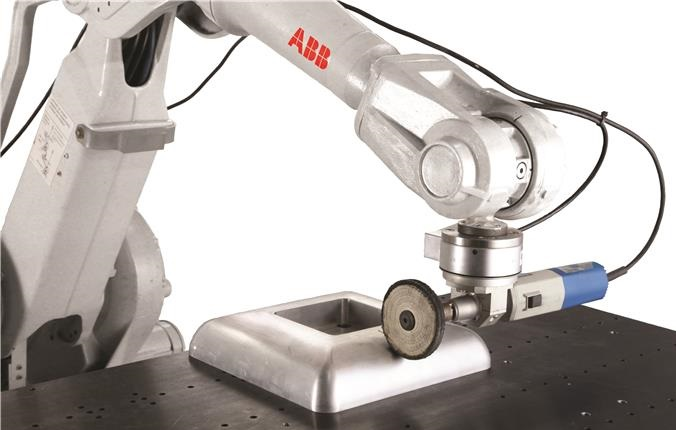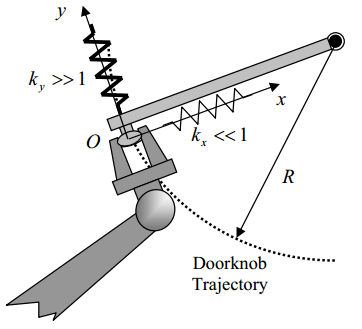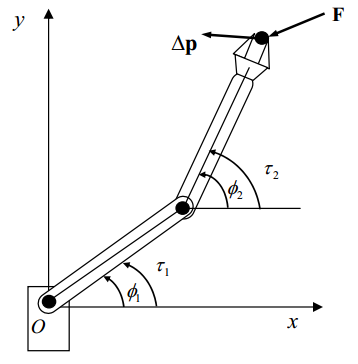# 机器人阻抗与导纳控制

目前用于喷漆搬运点焊等操作的工业机器人只具有简单的轨迹控制轨迹控制适用于机器人的末端执行器在空间沿某一规定的路径运动在运动过程中末端执行器不与任何外界物体接触对于执行擦玻璃转动曲柄拧螺丝研磨打毛刺装配零件等作业的机器人其末端执行器与环境之间存在力的作用且环境中的各种因素不确定此时仅使用轨迹控制就不能满足要求执行这些任务时必须让机器人末端执行器沿着预定的轨迹运动同时提供必要的力使它能克服环境中的阻力或符合工作环境的要求。以擦玻璃为例如果机器人手爪抓着一块很大很软的海绵并且知道玻璃的精确位置么通过控制手爪相对于玻璃的位置可以完成擦玻璃作业但如果作业是用刮刀刮去玻璃表面上的油漆而且玻璃表面空间位置不准确或者手爪的位置误差比较大由于存在沿垂直玻璃表面的误差作业执行的结果不是刮刀接触不到玻璃就是刮刀把玻璃打碎因此根据玻璃位置来控制擦玻璃机器人是行不通的比较好的方法是控制工具与玻璃之间的接触力这样即便是工作环境如玻璃位置不准确时也能保持工具与玻璃正确接触相应地机器人不但要有轨迹控制的功能而且要有力控制的功能。

下面是一个机器人开门的例子。如图所示，门把手的运动轨迹受到自然约束（机器人手爪与环境接触时，环境的几何特性构成对作业的约束），被限制在以转轴为圆心半径为R的圆弧上。传统的轨迹控制下为了保证机器人不把门或门把手扯掉，需要机器人沿着准确的轨迹运动，否则径向上很小的误差都会产生很大的力。如果只考虑静态，力和位置关系可用刚性矩阵来描述。如果考虑力和速度之间的关系，可用粘滞阻力矩阵来描述。通常对于需要进行位置控制的自由度，则要求在该方向上有很大的刚性，即表现出很硬的特性；对于需要力控制的自由度，则要求在该方向上有较小的刚性，即表现出较软的特性

对上面的问题，可以在径向赋予机器人较小的刚性（较大的柔性）。添加虚拟弹簧，将其刚度系数$k_x$设的很小，则当实际轨迹与期望轨迹不一致时弹簧产生的回复力也很小，不至于将门破坏掉。机器人末端与环境间的刚度矩阵可以定义为：$$\mathbf{K}=\begin{pmatrix}k_x & 0\\ 0 & k_y\end{pmatrix};k_x\ll 1,k_y\gg 1$$

通过雅可比矩阵可以将机器人末端刚度转换到关节空间中。设雅可比矩阵$\pmb{J}$将关节空间的速度$\dot{\pmb{q}}$映射到操作空间$\dot{\pmb{p}}$。末端刚度矩阵为$\pmb{K}_p$，$\Delta\pmb{p}$为末端位移变化量，$\pmb{F}$为末端位移变化引起的力。则有：$\pmb{F}=\pmb{K}_p\Delta\pmb{p}$

关节空间刚度矩阵$\mathbf{K}_q$可以根据雅可比矩阵计算出：$$\mathbf{K}_q=\mathbf{J}^T\mathbf{K}_p\mathbf{J}$$

简单推理过程为：$\pmb{\tau}=\pmb{J}^T\pmb{F}=\pmb{J}^T\pmb{K}_p\Delta\pmb{p}=\pmb{J}^T\pmb{K}_p\pmb{J}\Delta\pmb{q}$，根据$\pmb{\tau}=\pmb{K}_q\Delta\pmb{q}$，即可以推导出上面关系式。柔顺性分为主动柔顺性和被动柔顺性两类机器人凭借一些辅助的柔顺机构，使其在与环境接触时能够对外部作用力产生自然顺从，称为被动柔顺性。所谓被动柔顺机构，即利用一些可以使机器人在与环境作用时能够吸收或储存能量的机构器件如弹簧阻尼等构成的机构。机器人利用力的反馈信息采用一定的控制策略去主动控制作用力，称为主动柔顺性。

机器人的阻抗控制（Impedance Control）或导纳控制（Admittance Control）是通过调节机器人末端位置与力之间的动态特性来实现柔顺性。机器人与外界环境之间的交互可以由质量-弹簧-阻尼系统来描述，如下图所示，系统动力学方程为：$$m\ddot{x}+b\dot{x}+kx=f$$

其中$x$为位移，$m$为质量，$b$为阻尼，$k$为刚度，$f$为作用力对上面的动力学方程进行拉普拉斯变换，可以得到：$$(ms^2+bs+k)X(s)=F(s)$$

阻抗定义为$Z(s)=F(s)/X(s)$，导纳定义为阻抗的倒数：$Y(s)=Z^{-1}(s)=X(s)/F(s)$.由于$\Delta X=Y\Delta F$，如果导纳很小，那么力的变化$\Delta F$将产生很小的位移变化$\Delta X$。阻抗控制是一种建立在力与速度或位置之间的动态关系上的控制方法。通过建立机器人运动和外部作用力之间的动态关系，对机器人运动进行调节，从而实现机器人与环境的动态调整。$$M\ddot{x}+B\dot{x}+Kx=f_{ext}$$

对上面的方程有两种解读角度：

• 阻抗控制：当机器人末端产生位置偏差量$X$时，控制关节力矩使得其末端产生阻抗力$f_{ext}$
• 导纳控制：当机器人通过力/力矩传感器感知到外部作用力$f_{ext}$时，控制末端位移或速度

低阻尼参数可以提高机器人对操作者交互力的跟随能力，使得操作者可以通过较小的作用力就能使机器人跟随其做柔顺移动。但较低的阻尼参数却限制了拖动至目标点时交互的稳定性，机器人的位置会出现过冲通过调整 K，D 参数，可以改变实验特性，增加 K 时，单位作用力上产生的位移就变小，即需要用更大的力才能拉动关节；反之，减少 K 时，单位作用力上产生的位移就变大，用很小的力就能使关节产生较大的位移。增加 D 时，相当于增加系统的阻尼特性，可以进一步的提高系统运动时的平稳性，但如果 D 很大，可能出现系统响应很慢的情况，不能很好的跟随所施加的外力信息；另一方面，减少 D 时，可以适当的提高系统的快速性，但是过小的D 会引起系统的不稳定现象。

Force and Compliance Controls

Getting Started with Force Control

Robot Manipulator Control Theory and Practice

Impedance and Interaction Control - Summer School on Impedance

posted @ 2020-01-04 13:05  XXX已失联  阅读(7181)  评论(4编辑  收藏  举报